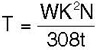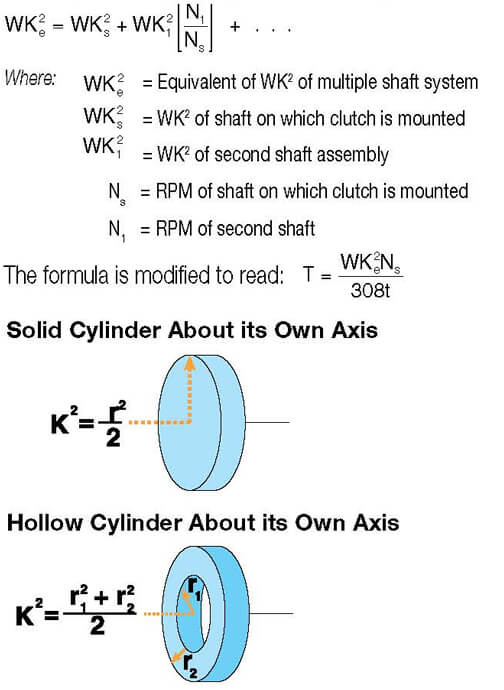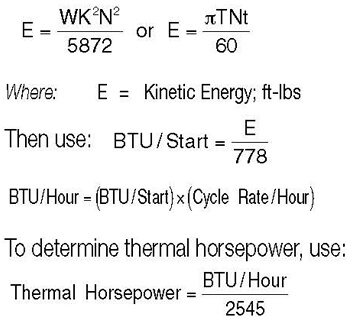# Disc Cone Clutch Application Worksheet

Use this form to request engineering assistance. The data you furnish will enable us to understand your application and recommend* the appropriate gearbox. When available, please attach prints or dimensional drawings.

*Recommendation is based on information supplied by the customer. Final acceptance and approval is the responsibility of the customer. Tolomatic recommends field testing or simulation of field testing on the machine it is designed for.

### Calculate Required Torque

Begin the calculation with this basic formula:Where:
T = Torque (in foot-pounds)
N = Speed (in RPM)
W = Weight of the Rotating Member (in pounds)
K2 = Radius of Gyration (in feet)
t = Starting time (in seconds)

The radius of gyration is the distance from the center of rotation at which the entire rotating mass could be concentrated and still be equivalent to the actual distributed mass (see axis diagrams).

For multiple shaft systems, use the following formula:### Calculate Heat Dissipation

Heat dissipation must also be considered in sizing a clutch. To find the amount of heat which an application will generate, which in turn must be dissipated, use the following formulae:Max. file size: 256 MB.
Max. file size: 256 MB.

### CONTACT INFORMATION

Name(Required)
Location(Required)